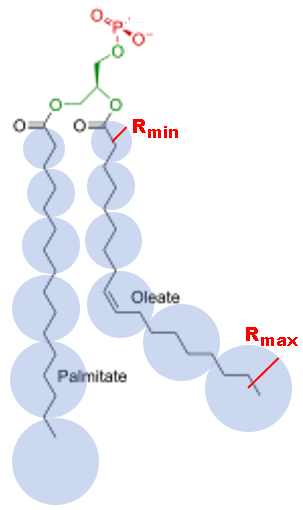$$\renewcommand\AA{\unicode{x212B}}$$

# EISFDiffSphereAlkyl¶

## Description¶This fitting function models the elastic incoherent intensity of a linear polycarbon chain (alkyl) where the local diffusion of each unit in the chain is modeled as undergoing continuous diffusion but confined to a spherical volume whose radius varies from a minimal value $$r$$ to a maximum value $$R$$ .

\begin{align}\begin{aligned}R_i = (R -r) * (i/M) + r\\EISF(Q) = \frac{1}{M} \sum_{i=1}^{M} (3 \frac{j_1(QR_i)}{QR_i})^2(Q\cdot R_i)\end{aligned}\end{align}

$$r$$ and $$R$$ units are inverse of $$Q$$ units. Because of the spherical symmetry of the problem, the structure factor is expressed in terms of the $$j_l(z)$$ spherical Bessel functions.

Related functions: - EISFDiffSphere - ElasticDiffSphere - InelasticDiffSphere - DiffSphere

## Usage¶

Example - fit of Q-dependence:

q =  [0.3, 0.5, 0.7, 0.9, 1.1, 1.3, 1.5, 1.7, 1.9]
# A=2.0, r=0.12, R=1.6, M=9
eisf = [1.96561499215648, 1.90667574712235, 1.82327109734092,
1.72075639596568, 1.60529662348532, 1.48322421137169,
1.36043655237770, 1.24191091785048, 1.13138963884055]
w = CreateWorkspace(q, eisf, NSpec=1)
results = Fit('name=EISFDiffSphereAlkyl, M=9', w, WorkspaceIndex=0)
print(results.Function)


Output:

name=EISFDiffSphereAlkyl,M=9,A=2,Rmin=0.12,Rmax=1.6


## Properties (fitting parameters)¶

Name

Default

Description

A

1.0

Amplitude

Rmin

1.0

Minimum radius, inverse units of Q.

Rmax

2.0

Maximum radius, inverse units of Q.

Categories: FitFunctions | QuasiElastic

## Source¶

Python: EISFDiffSphereAlkyl.py